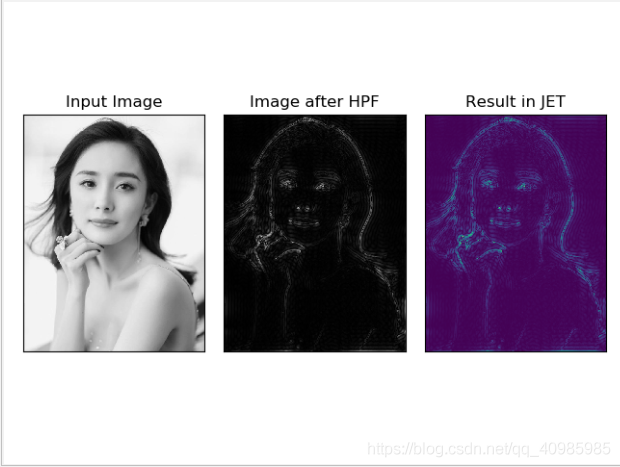﻿ OpenCV图像变换之傅里叶变换的一些应用_python_脚本之家
python# OpenCV图像变换之傅里叶变换的一些应用

## 前言

2D Discrete Fourier Transform (DFT)二维离散傅里叶变换
Fast Fourier Transform (FFT) 快速傅里叶变换

OpenCV使用cv2.dft()、cv2.idft() 实现傅里叶变换，效率更高一些（比OpenCV快3倍）

Numpy使用np.ifft2() 、np.fft.ifftshift() 实现傅里叶变换，使用更友好一些；

## 1. 效果图## 2. 原理

• DFT的性能优化：在一定的阵列尺寸下，DFT计算的性能较好。当数组大小为2的幂时，速度最快。大小为2、3和5的乘积的数组也可以非常有效地处理。

## 3. 源码

### 3.1 Numpy实现傅里叶变换

```# 傅里叶变换

import cv2
import numpy as np
from matplotlib import pyplot as plt

# 使用Numpy实现傅里叶变换：fft包
# fft.fft2() 进行频率变换
# 参数1：输入图像的灰度图
# 参数2：>输入图像 用0填充；  <输入图像 剪切输入图像； 不传递 返回输入图像
f = np.fft.fft2(img)

# 一旦得到结果，零频率分量（直流分量）将出现在左上角。
# 如果要将其置于中心，则需要使用np.fft.fftshift()将结果在两个方向上移动。
# 一旦找到了频率变换，就能找到幅度谱。
fshift = np.fft.fftshift(f)
magnitude_spectrum = 20 * np.log(np.abs(fshift))

plt.subplot(121), plt.imshow(img, cmap='gray')
plt.title('Input Image'), plt.xticks([]), plt.yticks([])
plt.subplot(122), plt.imshow(magnitude_spectrum, cmap='gray')
plt.title('Magnitude Spectrum'), plt.xticks([]), plt.yticks([])
plt.show()

# 找到了频率变换，就可以进行高通滤波和重建图像，也就是求逆DFT
rows, cols = img.shape
crow, ccol = rows // 2, cols // 2
fshift[crow - 30:crow + 30, ccol - 30:ccol + 30] = 0
f_ishift = np.fft.ifftshift(fshift)
img_back = np.fft.ifft2(f_ishift)
img_back = np.abs(img_back)

# 图像渐变章节学习到：高通滤波是一种边缘检测操作。这也表明大部分图像数据存在于频谱的低频区域。
# 仔细观察结果可以看到最后一张用JET颜色显示的图像，有一些瑕疵（它显示了一些波纹状的结构，这就是所谓的振铃效应。）
plt.subplot(131), plt.imshow(img, cmap='gray')
plt.title('Input Image'), plt.xticks([]), plt.yticks([])
plt.subplot(132), plt.imshow(img_back, cmap='gray')
plt.title('Image after HPF'), plt.xticks([]), plt.yticks([])
plt.subplot(133), plt.imshow(img_back)
plt.title('Result in JET'), plt.xticks([]), plt.yticks([])

plt.show()
```

### 3.2 OpenCV实现傅里叶变换

```import cv2
import numpy as np
from matplotlib import pyplot as plt

rows, cols = img.shape
print(rows, cols)

# 计算DFT效率最佳的尺寸
nrows = cv2.getOptimalDFTSize(rows)
ncols = cv2.getOptimalDFTSize(cols)
print(nrows, ncols)

nimg = np.zeros((nrows, ncols))
nimg[:rows, :cols] = img
img = nimg

# OpenCV计算快速傅里叶变换，输入图像应首先转换为np.float32，然后使用函数cv2.dft()和cv2.idft()。
# 返回结果与Numpy相同，但有两个通道。第一个通道为有结果的实部，第二个通道为有结果的虚部。
dft = cv2.dft(np.float32(img), flags=cv2.DFT_COMPLEX_OUTPUT)
dft_shift = np.fft.fftshift(dft)

magnitude_spectrum = 20 * np.log(cv2.magnitude(dft_shift[:, :, 0], dft_shift[:, :, 1]))

plt.subplot(121), plt.imshow(img, cmap='gray')
plt.title('Input Image'), plt.xticks([]), plt.yticks([])
plt.subplot(122), plt.imshow(magnitude_spectrum, cmap='gray')
plt.title('Magnitude Spectrum'), plt.xticks([]), plt.yticks([])
plt.show()

rows, cols = img.shape
crow, ccol = rows // 2, cols // 2

# 如何删除图像中的高频内容，即我们将LPF应用于图像。它实际上模糊了图像。
# 为此首先创建一个在低频时具有高值的掩码，即传递LF内容，在HF区域为0。
mask = np.zeros((rows, cols, 2), np.uint8)
mask[crow - 30:crow + 30, ccol - 30:ccol + 30] = 1

f_ishift = np.fft.ifftshift(fshift)
img_back = cv2.idft(f_ishift)
img_back = cv2.magnitude(img_back[:, :, 0], img_back[:, :, 1])

plt.subplot(121), plt.imshow(img, cmap='gray')
plt.title('Input Image'), plt.xticks([]), plt.yticks([])
plt.subplot(122), plt.imshow(img_back, cmap='gray')
plt.title('Magnitude Spectrum'), plt.xticks([]), plt.yticks([])
plt.show()
```

### 3.3 HPF or LPF？

```import cv2
import numpy as np
from matplotlib import pyplot as plt

# 简单的均值滤波
mean_filter = np.ones((3, 3))

# 构建高斯滤波
x = cv2.getGaussianKernel(5, 10)
gaussian = x * x.T

# 不同的边缘检测算法Scharr-x方向
scharr = np.array([[-3, 0, 3],
[-10, 0, 10],
[-3, 0, 3]])
# Sobel_x
sobel_x = np.array([[-1, 0, 1],
[-2, 0, 2],
[-1, 0, 1]])
# Sobel_y
sobel_y = np.array([[-1, -2, -1],
[0, 0, 0],
[1, 2, 1]])
# 拉普拉斯
laplacian = np.array([[0, 1, 0],
[1, -4, 1],
[0, 1, 0]])

filters = [mean_filter, gaussian, laplacian, sobel_x, sobel_y, scharr]
filter_name = ['mean_filter', 'gaussian', 'laplacian', 'sobel_x', \
'sobel_y', 'scharr_x']
fft_filters = [np.fft.fft2(x) for x in filters]
fft_shift = [np.fft.fftshift(y) for y in fft_filters]
mag_spectrum = [np.log(np.abs(z) + 1) for z in fft_shift]

for i in range(6):
plt.subplot(2, 3, i + 1), plt.imshow(mag_spectrum[i], cmap='gray')
plt.title(filter_name[i]), plt.xticks([]), plt.yticks([])

plt.show()

```

## 参考

• https://docs.opencv.org/3.0-beta/doc/py_tutorials/py_imgproc/py_transforms/py_fourier_transform/py_fourier_transform.html#fourier-transform